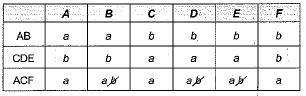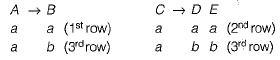Courses

# R = (A, B, C, D, E, F)R1 - (A, B)R2 = (C,D,E)R3 = (A, C, F)F= {A→B; C → DE ; AC → F;}​Does the above FD’s has lossless join property?a)Yesb)Noc)Data insufficientd)Cannot be determineCorrect answer is option 'A'. Can you explain this answer? Related Test: Functional Dependencies And Normalization (Advance Level)- 1

## Computer Science Engineering (CSE) Question

SANKHA SUVRA answered May 10, 2020
R = {A, B, C, D, E, F}
F = {A → B , C → DE, AC → F)
R1 = ( A B )
R2 =.{C,D,E}
R3 = {A, C, F}Convert b to a in 3rd row convert b to a in 3rd row. Since 3rd row contains all ‘a’s hence the relation has lossless join property.